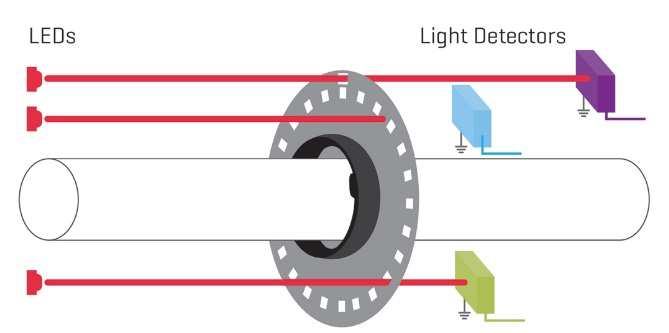# How does an optical Encoders works?### Introduction

The encoders use rotating discs with optical windows, as shown in the figure below. The encoder contains an optical disk with fine windows engraved on it. The light from the emitters goes through the openings in the disk to the detectors. When the encoder shaft is rotated, the light beams break. The encoder shown here is a quadrature encoding, and will be discussed below.There are two fundamental types of encoders; absolute and incremental. An absolute encoder will measure the position of the axis for a single rotation. The same axis angle will always produce the same reading. The output is usually a binary or gray code number. An incremental (or relative) encoder will emit two pulses that can be used to
determine the displacement. The logic circuits or software are used to determine the direction of rotation and count the pulses to determine the displacement. The speed can be determined by measuring the time between pulses.

The encoder discs are shown in the figure below. The absolute encoder has two rings, the outer ring is the most significant digit of the encoder, the inner ring is the least significant digit. The relative encoder has two rings, with one ring rotated a few degrees in front of the other, but otherwise the same. Both rings detect position to a quarter of the disc. To add accuracy for the absolute encoder More rings must be added to the disk and more transmitters and detectors. To add precision to the relative encoder, we only need to add more windows to the two existing rings. Typical encoders will have from 2 to thousands of windows per ring.When using absolute encoders, the position during a single rotation is measured directly. If the encoder rotates multiple times then the total number of rotations must be counted separately.

When using a relative encoder, the distance of rotation is determined by counting the pulses from one of the rings. If the encoder only rotates in one direction then a simple count of pulses from one ring will determine the total distance. If the encoder can rotate both directions a second ring must be used to determine when to subtract pulses. The quadrature scheme, using two rings, is shown in Figure below. The signals are set up so that one is out of phase with the other. Notice that for different directions of rotation, input B either leads or lags A.